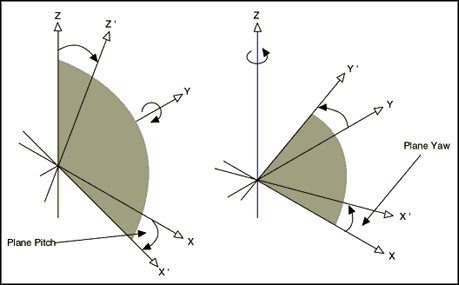# Spherical Interpolation

Publish Date: Sep 06, 2016 | 0 Ratings | 0.00 out of 5 | Print

Spherical interpolation is a special feature available in FlexMotion that causes a vector to move in a path along the outside of a sphere.

Parameters that must be specified when performing spherical interpolation are as follows:

Radius is the arc radius in counts (servo axes) or steps (stepper axes). The range is 2 to (2^31) - 1 counts (steps).

Start Angle is the double precision floating point value in degrees of the starting angle of the arc. The range is 0 to 360°, where angle 0 is along the positive X’ axis and values increase counter-clockwise from the positive X’ axis in the X’Y’ plane.

Travel Angle is the double precision floating point value in degrees of the angle to be traversed. The range is -1,474,560 to +1,474,200° (-4,096 to +4,095 revolutions). A positive travel angle defines counter-clockwise rotation in the X’Y’ plane.

Plane Pitch is the double precision floating point value in degrees of the angle between the X’ and X axes when the entire X’Y’Z’ vector space is rotated around the Y axis. The Y’ axis remains aligned with the Y axis. The range is 0 to 90°. When the plane pitch equals 90°, the positive X’ axis is aligned with the negative Z axis.

Plane Yaw is the double precision floating point value in degrees of the angle between the X’ and X axes when the entire X’Y’Z’ vector space is rotated around the Z axis. The Z’ axis remains aligned with the Z axis. The range is 0 to 360°. When the plane yaw equals 90°, the positive X’ axis is aligned with the positive Y axis.

The Load Spherical Arc function/VI defines an arc in the X’Y’ plane of a coordinate system that has to be transformed by rotation in pitch and yaw from the normal 3D vector space (XYZ). In the transformed X’Y’Z’ space, the spherical arc is reduced to a simpler circular arc. It is specified by a radius, starting angle, and travel angle, and, like all vector space moves, uses the loaded value of vector acceleration and vector velocity to define the motion along the path of the arc in the X’Y’ plane.

The following figure shows a graphic representation of the transformation between the C and XYZ coordinate spaces. The formal definitions of Plane Pitch and Plane Yaw are described above.There are several things you should keep in mind when using spherical interpolation. Pitch and yaw transformations are inherently confusing because they interact with each other. To avoid ambiguities, you can think about spherical arcs and coordinate transformations as follows:

• The spherical arc is defined as a circular arc in the X’Y’ plane of a transformed vector space X’Y’Z’. The original vector space XYZ is defined by the Configure Vector Space function/VI.
• The transformed vector space X’Y’Z’ is defined in orientation only, with no absolute position offset. Its orientation is with respect to the XYZ vector space and is defined in terms of pitch and yaw angles.
• Pitch angle rotation comes before yaw angle rotation.
• When rotating through the pitch angle, the Y and Y’ axes stay aligned with each other while the X’Z’ plane rotates around them.
• When rotating through the yaw angle, the Y’ axis never leaves the original XY plane as the newly defined X’Y’Z’ vector space rotates around the original Z axis.
• At the beginning of the move, the axes are considered to be already on the arc in the X’Y’ plane. This avoids any impossible situations where the end point of the last move and the beginning of the arc move are not coincident.

Spherical arcs are one of the most powerful, unique and, unfortunately, complex features of FlexMotion. They allow full 3D curvilinear motion for robotics, solid modeling, and other advanced applications.

### Ratings

Rate this document

Answered Your Question?
Yes No